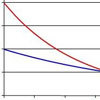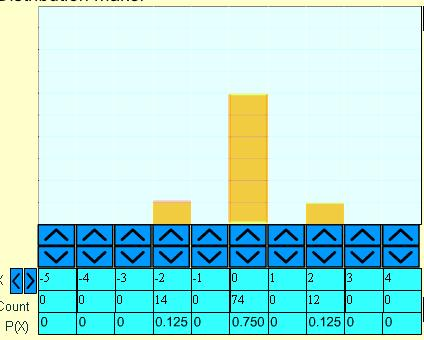#### You may also like### Spinners

How do scores on dice and factors of polynomials relate to each other?### Data Matching

Use your skill and judgement to match the sets of random data.### Into the Exponential Distribution

Get into the exponential distribution through an exploration of its pdf.

# Random Inequalities

##### Age 16 to 18Challenge Level

James Bell from the MacMillan academy was the first person to crack this toughnut, with this solution. He also noted that the problem as it stood only held for positive random variables -- well spotted. Well dones James!

For the first part James cleverly used random variables which only took a single value to work out that $??$ must equal $1$:

Part 1:

Consider $X$ such that $P(X=10)=1$ $E(|X|)=10$ taking $a=9$ we get left hand side equal to $1$ so the right handside must equal at least $1 =\frac{10}{9^{??}}$. If $??$ were $2$ or greater this wouldn't be satisfied.

Now consider $P(X=0.5)=1$ so $E(|X|)=0.5$. Taking $a=0.45$ we get left hand side equal to $1$ so the right handside must equal at least $1 =\frac{0.5}{0.45^{??}}$. If $??$ were $0$ or less this wouldn't be satisfied and so $??=1$.

Part 2:

Our task is to design a distribution such that the chance of $X$ being at least $2\sigma$ from the mean is maximised.

James first argues that a distribution which satisfied the inquality exactly must have a maximum value of $2\sigma$ by shifting probability around. Some more details would be needed for a fully convincing explanation, but the argument is in essence correct and shows very sophisticated statistical reasoning .

If a solution existed where $X$ could take a value greater than $2\sigma$ from the mean then we could use this to create a new distribution $Y$ by changing the values of events leading to a difference being greater than $2\sigma$ to values leading to a difference of $2\sigma$, thus making the probability of the difference being greater than $2\sigma$ zero. Of course, this would decrease $2\sigma$ for $Y$; some of the probability for $Y$ could be moved from near the mean to outside $2\sigma$ for $Y$ without moving $2\sigma$ for $Y$ as far out to where it was originally for $X$, meaning that the probability of $|Y-\mu|$ being greater than $2\sigma$ would increase above $\frac{1}{4}$. As we know this to be impossible, any such distribution must have $P(|X-\mu|> 2\sigma)=0$.

With this insight, James was able to search for a solution

Assuming symmetry, to find a solution let us set
$$P(X-\mu=2\sigma)=P(\mu-X=2\sigma)=0.125\,,$$
as together they must add to $0.25$ or $\frac{1}{4}$ the other $\frac{3}{4}$ must be distributed right on the mean in order to makle the variance as small as possible which suggests the distribution attached for $\sigma=1\,, \mu=0$, and sure enough calculating sigma from the distribution gives $\sigma=1$ as well confirming that it works.

Here is the picture: